Skip to main content

# Generalized function projective synchronization of chaotic systems for secure communication

## Abstract

By using the generalized function projective synchronization (GFPS) method, in this paper, a new scheme for secure information transmission is proposed. The Liu system is employed to encrypt the information signal. In the transmitter, the original information signal is modulated into the system parameter of the chaotic systems. In the receiver, we assume that the parameter of receiver system is uncertain. Based on the Lyapunov stability theory, the controllers and corresponding parameter update rule are constructed to achieve GFPS between the transmitter and receiver system with uncertain parameters, and identify unknown parameters. The original information signal can be recovered successfully through some simple operations by the estimated parameter. Furthermore, by means of the proposed method, the original information signal can be extracted accurately in the presence of additional noise in communication channel. Numerical results have verified the effectiveness and feasibility of presented method.

Mathematics subject classification (2010)

68M10, 34C28, 93A30, 93C40

## 1 Introduction

Chaos is a kind of characteristics for nonlinear systems, which is a bounded unstable dynamic behavior that exhibits sensitive dependence on initial conditions and includes infinite unstable periodic motions. Since Pecora and Carroll  presented the conception of chaotic synchronization for two identical chaotic systems with different initial conditions, many synchronization methods have been proposed, such as complete synchronization (CS) , generalized synchronization , phase synchronization , impulse synchronization , lag synchronization , projective synchronization , etc. Amongst all kinds of chaos synchronization, projective synchronization, first reported by Mainieri and Rehacek , has been especially extensively studied because it can obtain faster communication with its proportional feature .

However, the above projective synchronization (PS) method is characterized that its drive and response systems are synchronized up to a constant scaling factor. Recently, Chen et al.  introduced a new PS scheme which is called function projective synchronization (FPS), where the responses of synchronized dynamical states can synchronize up to a scaling function factor. Let the scaling function be constant or unity, one can obtain PS or CS. So FPS is a more general definition of PS. Be-cause the unpredictability of the scaling function in FPS can additionally enhance security of communication, this feature could be applied to get more secure communication. More recently, many studies concentrate on FPS of chaotic systems and its application to secure communication . For instance, FPS of two identical or different chaotic systems was studied in [13, 14, 16, 18]. In [15, 20], another new synchronization phenomenon, generalized function projective synchronization (GFPS), was proposed, in which drive and response systems could be synchronized to a scaling function matrix. In , Yu and Li investigated GFPS of two entirely different systems with fully unknown parameters. Du et al.  studied GFPS in coupled chaotic systems and its application in secure communication.

In the past decades, the use of chaotic signals for information transmission attracts great attention of modern scientists from various fields [16, 17, 2026]. Different approaches for transmission of information signals using chaotic dynamics have been proposed, such as chaotic masking, chaotic modulation, nonlinear mixing, chaotic switching, and others. In a typical chaotic synchronization communication scheme, the information to be transmitted is carried from the transmitter to the receiver by a chaotic signal through an analog channel. In the receiver, chaos synchronization is employed to recover the information signal. In many existing secure communication methods, the information signal is directly added to input of chaotic systems. The magnitude of transmitted signals is required to be sufficiently small, otherwise it may lead to the instability of whole system. On the other hand, although these communication methods have been successfully demonstrated in simulations, performance of communication schemes were usually quantified by assuming the identical chaos synchronization based on exact knowledge of the system parameters [27, 28], which may impose some limitations to applicability of these techniques. But in real situation, some or all of parameters are unknown and the noise exists. The effect of these uncertainties and noise will destroy the synchronization and even break it. As a result, one cannot extract the original information in the receiver. Therefore, it is essential to study secure communication in the presence of unknown parameters and noise.

Motivated by the above discussions, this paper proposes a new secure communication scheme based on GFPS of uncertain Liu chaotic system and parameter modulation. In the transmitter, the original information signal is firstly transformed by an invertible function. Then the processed signal is modulated into the parameter of Liu system. The resulting system is still chaotic. In our method, no constraint is imposed on the magnitude of the original information signal. Suppose the parameter of the receiver system is unknown. Based on the Lyapunov stability theory, we design the controllers and the parameter update rule to realize GFPS of uncertain Liu chaotic systems and identify the unknown parameter of the receiver system. Then the information signal in the receiver can be recovered by the estimated parameter. Moreover, it is worth noting that secure communication using GFPS can still be realized fast even if the transmission channel is perturbed by additive noise.

The rest of this paper is organized as follows. In Section 2, Liu system is described briefly and the definition of GFPS is presented. Section 3 gives the chaotic secure communication scheme using GFPS and parameter modulation. By means of the Lyapunov stability theory and adaptive control, the controllers and corresponding parameter update rule are designed to ensure GFPS between two identical Liu chaotic systems with uncertain parameters. Simulation experiments of the proposed secure communication system have been performed in Section 4. The conclusions are finally drawn in Section 5.

## 2 System description and the definition of GFPS

### 2.1 The Liu system

In 1963, Lorenz  found the first classical chaotic attractor in a three-dimensional autonomous system derived form a simplified model of earth atmospheric convection system. As the first chaotic model, the Lorenz system has become a paradigm of chaos research. Mathematicians, physicists and engineers from various fields have thoroughly studied the essence of chaos, characteristics of chaotic systems, bifurcations, routes to chaos, and many other related topics. There are also some chaotic systems of great significance that are closely related to the Lorenz system but not topologically equivalent to it, such as the Rössler system , the Chen system  and the Lü system . Recently, Liu et al.  proposed a system of three-dimensional autonomous differential equations with only two quadratic terms, which is described as follows: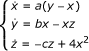(1)

where (x, y, z) R3 are state variables, (a, b, c) R3 are all positive real parameters and c varies in a certain range. In the Liu system, when the parameter c varies in a big range, the resulting system can still be chaotic and have more abundant and complex behaviors than the original Liu system. And compared to other parameters, the parameter c is more suitable to be used in parameter modulation. Figure 1 displays the Lyapunov exponents spectrum of system (1) with a = 10, b = 40 and c [0,8]. Obviously, when c [0.5, 8], system (1) is always chaotic. The chaotic attractors of Liu system with different c are shown in Figure 2.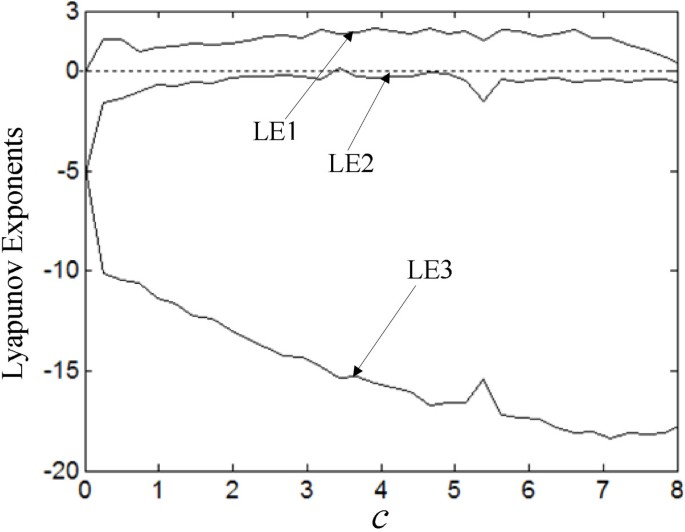Figure 1Figure 2

The Liu system has a butterfly-shaped attractor similar to the Lorenz attractor but not equivalent. The existing studies have shown that the Liu system is equivalent to Shimizu-Morioka system and is a representative example of chaotic attractors. Compared to other chaotic systems, when the system parameters of Liu system vary in a certain range, this chaotic system can still be chaotic, further the dynamical behaviors of this system become more abundant and complex. Therefore, it is possible to modulate information signal into the parameter of chaotic systems to realize chaotic secure communication.

Remark 1 It is found that many chaotic systems can maintain chaotic behavior when a system parameter continuously changes in a certain range. We can obtain a "new" system parameter by modulating the information signal into the parameter of chaotic systems. If the "new" system parameter can ensure the corresponding system still be chaotic, the detector cannot extract the information signal from the signals, and transmitted in the channel. So it is possible to modulate the information signal into the parameter of chaotic systems to realize chaotic secure communication.

### 2.2 The definition of GFPS

The drive system and the response system are defined as follows: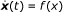(2)(3)

where x= (x1, x2, ..., x n ) Rn, y= (y1, y2, ..., y n ) Rn are the state vectors; f, g : RnRn are differentiable functions; U(x, y) is a controller to be designed. Let us define the error vector as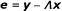(4)

where e= (e1, e2, ..., e n )T , and Λ= diag(ϕ1(t), ϕ2t, ..., ϕ n (t)) is reversible and differentiable, where ϕ i (t): RnR(≠ 0) is a continuously differentiable functions with bounded.

Definition 1 (GFPS) For the drive system (2) and the response system (3), it is said that system (2) and (3) are GFPS, if there exists a scaling function matrix Λ such that limt→∞||e|| = 0.

Remark 2 We call Λ a scaling function matrix and ϕ i (t) a scaling function factor, respectively. It is easy to see that if ϕ1(t) = ϕ2(t) = = ϕ n (t), GFPS is simplified to FPS. If ϕi = a i (i = 1, 2, ..., n) where a i R and a i ≠ 0, GPS will occur. If Λ = λI where λ R is a constant and I n is an n × n identity matrix, GFPS is reduced to PS. In particular, if ϕ i (t) = 1 or ϕ i (t) = -1, the problem further becomes standard CS or anti-synchronization (AS).

## 3 Secure communication based on GFPS and parameter modulation

### 3.1 The chaotic secure communication scheme using GFPS and parameter modulation

The secure communication system involves the development of a signal that contains the information which is to remain undetectable by others within a carrier signal. We can ensure the security of this information by inserting it into a chaotic signal which is transmitted to a prescribed receiver that would be able to detect and recover the information from the chaotic signal.

In the present application, we propose a new chaotic secure communication scheme using GFPS of uncertain Liu chaotic system and parameter modulation, as shown in Figure 3. The system consists of a transmitter module, a communication channel and a receiver module. In this scheme, the chaotic signal is generated by using Liu chaotic system which was described by the system model of differential equation (1). For convenience, the chaotic system is written as the following form:Figure 3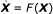(5)

where the state vector X= (x, y, z).

The transmitter module is composed of a chaotic system S1 and an invertible transformation function φ. The "new" parameter β is formulated as a function of the original information signal f (t), i.e., β = φ(f (t)), which meets the need of the parameter range of c. So the resulting system still exhibits a chaotic behavior. In our method, the following two cases: no circumstance noise and additive noise in the transmission channel will be considered. The receiver module consists of another chaotic system S2, the controller U(S1, S2) and the corresponding inverse function φ-1. Assume that the parameter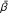of the receiver system S2 is uncertain. In the receiver, with the help of controller U(S1, S2), GFPS between systems S1 and S2 can be realized. The unknown parametercan be asymptotically identified as GFPS appears. Then the original in-formation can be recovered through some simple operations by the function φ-1 and the estimated parameter, i.e.,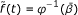.

### 3.2 GFPS of uncertain Liu chaotic system

As we all know, the information signal is often bounded; in other words, the information signal f (t) satisfies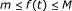(6)

where m and M are known constants.

For the Liu system, we will employ the parameter to transmit the information signal. Define a "new" parameter β as follows:(7)

Obviously, the "new" parameter satisfies β [0.5, 8]. According to the above description of Liu system, we know the following system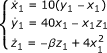(8)

will still be chaotic, which has more abundant dynamic behavior. Since the resulting system (8) is chaotic, it is hard to detect information signal from the signals transmit-ted in the channel.

We take the system given by Equation (8) as the transmitter system and the receiver system is constructed as follows: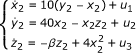(9)

where u i (t) (i = 1, 2, 3) are controllers to be designed,is unknown parameter of receiver system which needs to be estimated.

Define the GFPS error variables as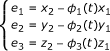(10)

and denote the parameter estimate error as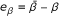(11)

The time derivative of the error variables (10) and (11) is: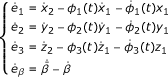(12)

Subtracting Equation (8) from Equation (9) yields the following error dynamical system: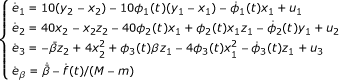(13)

Our aim is to find appropriate controllers u i (t) (i = 1, 2, 3) for stabilizing error variables at the origin, i.e., limt→∞||e|| = 0 and limt→∞e β = 0 where e= (e1, e2, e3)T . That is, GFPS between the transmitter system (8) and the receiver system (9) are globally achieved and the uncertain parameter can be identified asymptotically. To this end, we design controllers as follows: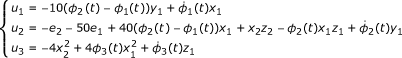(14)

and the update rule for unknown parameter as below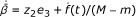(15)

Then we have the following main theorem.

Theorem 1 For given nonzero scaling functions ϕ i (t)(i = 1, 2, 3), GFPS between the transmitter system (8) and the receiver system (9) can occur by the controllers (14) and the parameter update rule (15). It implies that the GFPS errors satisfy limt→∞e i (t) = 0. The uncertain parameter is well estimated from the system parameter in the sense of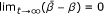.

Proof We choose the following Lyapunov function for the error system (13):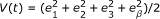(16)

Taking the time derivative of V(t) along the trajectories of the error system (13) yields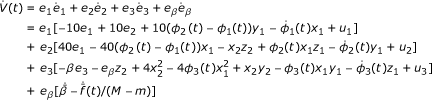(17)

Substituting Equations (14) and (15) into Equation (17), one can obtain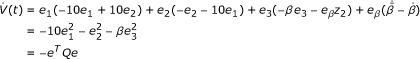where Q = diag(10, 1, β ) and β [0.5, 8]. Clearly, Q is a positive definite matrix and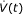is negative definite. Based on the Lyapunov stability theory, the error dynamical system (13) is globally and asymptotically stable at the origin, and we have e β → 0, as t. Hence GFPS between the transmitter system (8) and the receiver system (9) is achieved and the uncertain parameter is also identified in the receiver end simultaneously under the controllers (14) and the parameter update rule (15). The proof is completed.

When GFPS between the transmitter system and the receiver system appear, ac-cording to Theorem 1, the uncertain parametercan be estimated asymptotically, i.e.,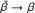. So the original information signal can be recovered in the receiver as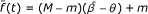(18)

where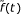denotes the recovered signal. Thus we have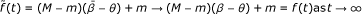which implies that the original information signal can be recovered successfully in the receiver end.

Remark 3 In practical situations, if the information signal to be transmitted is too large, it will result in a chaotic system to be asymptotically stable or emanative. In this case, one may fail to extract the information signal. In the presented method, no constraint is imposed on the information signal. The original information signal is firstly transformed by an invertible function. Then the transformed signal is used as the parameter of Liu system. The resulting system exhibits more abundant chaotic behavior. The interceptor cannot extract the information from the transmitted signals in the channel.

## 4 Simulation results

To verify and demonstrate the effectiveness of the proposed chaotic secure communication scheme, some numerical examples are performed in this section. In the simulations, the ODE45 algorithm is applied to solve the differential systems. The initial states of the transmitter system (8) and the receiver system (9) are arbitrarily taken as x1(0), y1(0), z1(0) = (2, 1, - 1) and (x2(0), y2(0), z2(0)) = (-3, -2, 4), respectively. The initial values of the unknown parameter is chosen arbitrarily as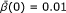.

### 4.1 Secure communication without circumstance noise

In this section, we focus on considering the case of no circumstance noise in the transmission channel. We choose the original information signal as f (t) = 1.5 + 2.5 sin(2t). The scaling functions are selected arbitrarily as ϕ1(t) = 0.5 sin t+2, ϕ2(t) = 3 cos(2 * t) - 1 and ϕ3(t) = - 1.5. It is obvious that -1 ≤ f (t) ≤ 4. Let θ = 2, by Equation (7), we obtain the "new" parameter as β = 0.5 sin(2t) + 2.5. Clearly, β [2, 3]. Therefore, the resulting system (8) is still chaotic, as depicted in Figure 4. So one cannot extract the information signal from the transmitted signals x1, y1 and z1. The simulation results of the proposed secure communication scheme using GFPS between systems (8) and (9) by the controllers (14) and the parameter update rule (15) are shown in Figure 5. Figure 5 plots the original information signal f (t). Figure 5 displays the time evolution of the GFPS errors, which shows that the time response of the GFPS errors e i (t)(i = 1, 2, 3) converge to zero after t > 0.4. The recovered signalis shown in Figure 5 and and the error signal between the original information signal and the recovered one is plotted in Figure 5. From Figure 5, one can easily see that the original information signal f (t) is recovered accurately after t > 0.4.Figure 4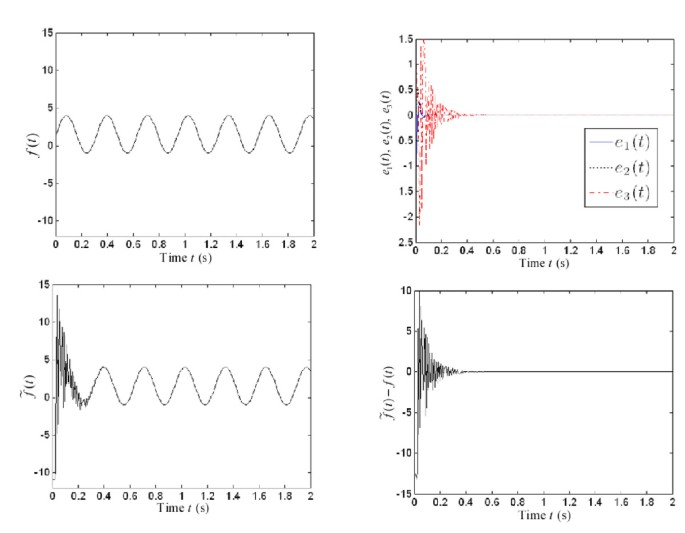Figure 5

The information signal f (t) and the scaling functions ϕ i (t) are chosen as those above. Set θ = 4. Then the "new" parameter β = 0.5 sin(2t) + 4.5. Obviously, β [4, 5]. Figure 6 displays that the resulting system (8) exhibits chaotic behavior. The numerical results of the proposed secure communication scheme are illustrated in Figure 7. Figure 7 shows that the synchronization errors e i (t) have been stabilized at the origin after t > 0.25, which implies that GFPS between the transmitter system (8) and the receiver system (9) is achieved. The original information signal and the recovered one are depicted in Figure 7. Figure 7 displays the error signal between the original information signal and the recovered one, from which it is found that the original information signal f (t) can be extracted exactly after t > 0.25. Figure 8 describes the logarithm of the absolute value of the error signal when β = 0.5 sin(2t) + 2.5 and β = 0.5 sin(2t) + 4.5, respectively. From Figure 8, we find that the synchronization rate when β = 0.5 sin(2t) + 4.5 is faster than that when β = 0.5 sin(2t) + 2.5, and the recovered signal when β = 0.5 sin(2t) + 4.5 is more accurate than that when β = 0.5 sin(2t) + 2.5.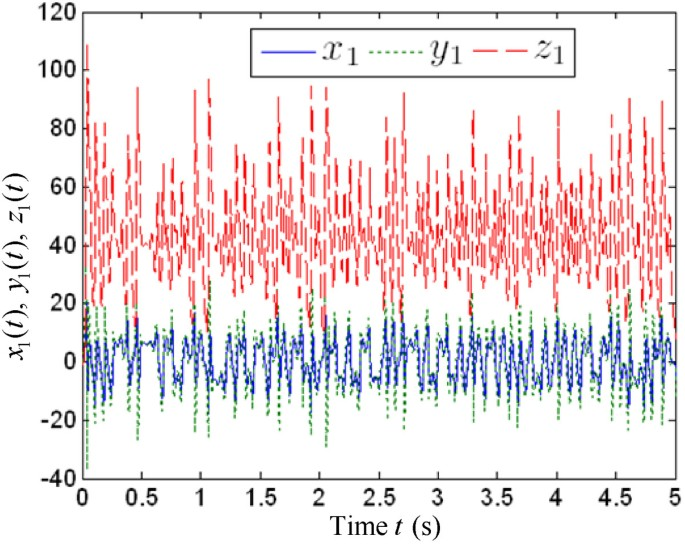Figure 6Figure 7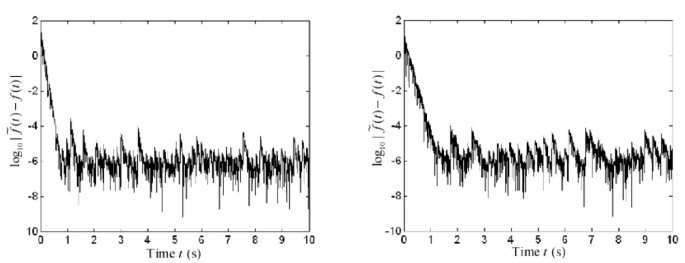Figure 8

Similarly, we have also tested the proposed secure communication scheme for other values of θ. Limited to the length of this paper, we omit these results here. Numeric evidence shows that the larger the value of θ, the faster to achieve GFPS, and the more accurate the recovered signal is.

### 4.2 Secure communication with additive noise

Here, we will study the proposed chaotic secure communication scheme when there exists additive noise in the channel. Suppose the additive noise N(t) = (0.01 sin(10t), 0.02 sin(20t), 0.03 sin(30t)). Choose the information signal as f (t) = 4 cos(0.5t) - 3. The scaling functions are selected randomly as ϕ1(t) = 1 + e-t, ϕ2(t) = 2.5 + 3 sin(0.5 * t) and ϕ3(t) = 1.2 + 0.3 cos(10t). Take θ = 3. Thus the "new" parameter β = 0.5 cos(0.5t) + 3.5 and β [3, 4]. So the resulting system (8) is still chaotic, as depicted in Figure 9. The simulation results of the proposed chaotic secure communication scheme with additive noise are displayed in Figure 10. The synchronization errors e1, e2 and e3 are shown in Figure 10, from which we can see that the required synchronization has been achieved quickly with our designed controllers (14) and the parameter update rule (15). The information signal f (t) and the recovered oneare displayed in Figure 10. Figure 10 plots the error signal between the original information signal and the recovered one. From Figure 10, it is easy to see that the information signal f (t) is recovered accurately after a very short transient.Figure 9Figure 10

## 5 Conclusions

In this paper, a novel chaotic secure communication scheme based on GFPS and parameter modulation is proposed. In the transmitter end, the original information signal is modulated into the parameter of the chaotic system and the resulting system still chaotic. There is no constraint posed on the original information signal. In the receiver, we assume that the parameter of the receiver system is uncertain. On the basis of the Lyapunov stability theory, the controllers and corresponding parameter update rule are devised to make the states of two identical Liu chaotic systems with unknown parameter synchronized. Simultaneously the uncertain parameter of the receiver system is also identified. Furthermore, the information signal can also be recovered accurately and fast by applying our secure communication method when additive noise exists in the transmission channel. Numerical simulations show the effectiveness and feasibility of the proposed chaotic secure communication scheme based on GFPS and parameter modulation.

## References

1. 1.

Pecora LM, Carroll TL: Synchronization in chaotic systems. Phys Rev Lett 1990,64(8):821-824. 10.1103/PhysRevLett.64.821

2. 2.

Ge Z, Chang C: Generalized synchronization of chaotic systems by pure error dynamics and elaborate Lyapunov function. Nonlinear Anal Theory Methods Appl 2009,71(11):5301-5312. 10.1016/j.na.2009.04.020

3. 3.

Breve FA, Zhao L, Quiles MG, Macau EEN: Chaotic phase synchronization and desynchronization in an oscillator network for object selection. Neural Netw 2009,22(5-6):728-737. 10.1016/j.neunet.2009.06.027

4. 4.

Ren Q, Zhao J: Impulsive synchronization of coupled chaotic systems via adaptive-feedback approach. Phys Lett A 2006,355(4-5):342-347. 10.1016/j.physleta.2006.02.053

5. 5.

Li C, Liao X, Wong K: Chaotic lag synchronization of coupled time-delayed systems and its applications in secure communication. Physica D Nonlinear Phenom 2004,194(3-4):187-202. 10.1016/j.physd.2004.02.005

6. 6.

Mainieri R, Rehacek J: Projective synchronization in three-dimensional chaotic systems. Phys Rev Lett 1999,82(15):3042-3045. 10.1103/PhysRevLett.82.3042

7. 7.

Xu D: Control of projective synchronization in chaotic systems. Phys Rev E 2001, 63: 27201-27204.

8. 8.

Xu D, Chee CY: Controlling the ultimate state of projective synchronization in chaotic systems of arbitrary dimension. Phys Rev E 2002,66(4):046218-046222.

9. 9.

Chee CY, Xu D: Secure digital communication using controlled projective synchronization of chaos. Chaos Soliton Fract 2005,23(3):1063-1070.

10. 10.

Chen J, Jiao L, Wu J, Wang X: Projective synchronization with different scale factors in a driven-response complex network and its application in image encryption. Nonlinear Anal Real World Appl 2010,11(4):3045-3058. 10.1016/j.nonrwa.2009.11.003

11. 11.

Hoang TM, Nakagawa M: A secure communication system using projective-lag and/or projectiveanticipating synchronizations of coupled multidelay feedback systems. Chaos Soliton Fract 2008,38(5):1423-1438. 10.1016/j.chaos.2008.02.008

12. 12.

Li Z, Xu D: A secure communication scheme using projective chaos synchronization. Chaos Soliton Fract 2004,22(2):477-481. 10.1016/j.chaos.2004.02.019

13. 13.

Chen Y, Li X: Function projective synchronization between two identical chaotic systems. Int J Mod Phys C 2007,18(5):883-888. 10.1142/S0129183107010607

14. 14.

Du H, Zeng Q, Wang C: Function projective synchronization of different chaotic systems with uncertain parameters. Phys Lett A 2008,372(33):5402-5410. 10.1016/j.physleta.2008.06.036

15. 15.

Du H, Zeng Q, Wang C: Modified function projective synchronization of chaotic system. Chaos Soliton Fract 2009,42(4):2399-2404. 10.1016/j.chaos.2009.03.120

16. 16.

Du H, Zeng Q, Wang C, Ling M: Function projective synchronization in coupled chaotic systems. Nonlinear Anal Real World Appl 2010,11(2):705-712. 10.1016/j.nonrwa.2009.01.016

17. 17.

Du H, Zeng Q, Lü N: A general method for modified function projective lag synchronization in chaotic systems. Phys Lett A 2010,374(13-14):1493-1496. 10.1016/j.physleta.2010.01.058

18. 18.

Luo R: Adaptive function projective synchronization of Rösler hyperchaotic system with uncertain parameters. Phys Lett A 2008,372(20):3667-3671. 10.1016/j.physleta.2008.02.035

19. 19.

Sudheer KS, Sabir M: Adaptive function projective synchronization of two-cell Quantum-CNN chaotic oscillators with uncertain parameters. Phys Lett A 2009,373(21):1847-1851. 10.1016/j.physleta.2009.03.052

20. 20.

Yu Y, Li H: Adaptive generalized function projective synchronization of uncertain chaotic systems. Nonlinear Anal Real World Appl 2010,11(4):2456-2464. 10.1016/j.nonrwa.2009.08.002

21. 21.

Chen G, Dong X: From Chaos to Order: Methodologies, Perspectives and Applications. World Scientific, Singapore; 1998.

22. 22.

Kocarev L, Parlitz U: General approach for chaotic synchronization with applications to communication. Phys Rev Lett 1995,74(25):5028-5031. 10.1103/PhysRevLett.74.5028

23. 23.

Lin J-S, Huang C-F, Liao T-L, Yan J-J: Design and implementation of digital secure communication based on synchronized chaotic systems. Digital Signal Process 2010,20(1):229-237. 10.1016/j.dsp.2009.04.006

24. 24.

Moskalenko OI, Koronovskii AA, Hramov AE: Generalized synchronization of chaos for secure communication: remarkable stability to noise. Phys Lett A 2010,374(29):2925-2931. 10.1016/j.physleta.2010.05.024

25. 25.

Wu X: A new chaotic communication scheme based on adaptive synchronization. Chaos: An Inter-disciplinary J Nonlinear Sci 2006,16(4):043118. 10.1063/1.2401058

26. 26.

Zhu F: Observer-based synchronization of uncertain chaotic system and its application to secure communications. Chaos Soliton Fract 2009,40(5):2384-2391. 10.1016/j.chaos.2007.10.052

27. 27.

Andrievsky B: Adaptive synchronization methods for signal transmission on chaotic carriers. Math Comput Simul 2002,58(4-6):285-293. 10.1016/S0378-4754(01)00373-1

28. 28.

Bai EW, Lonngren KE: A Ucar, Secure communication via multiple parameter modulation in a delayed chaotic system. Chaos Soliton Fract 2005,23(3):1071-1076.

29. 29.

Lorenz EN: Deterministic nonperiodic flow. J Atmos Sci 1963, 20: 130-141. 10.1175/1520-0469(1963)020<0130:DNF>2.0.CO;2

30. 30.

Rösler OE: An equation for continuous chaos. Phys Lett A 1976,57(5):397-398. 10.1016/0375-9601(76)90101-8

31. 31.

Chen G, Ueta T: Yet another chaotic attractor. Int J Bifurc Chaos 1999,9(7):1465-1466. 10.1142/S0218127499001024

32. 32.

Lü J, Chen G, Zhang S: The compound structure of a new chaotic attractor. Chaos Soliton Fract 2002,14(5):669-672. 10.1016/S0960-0779(02)00007-3

33. 33.

Liu C, Liu T, Liu L, Liu K: A new chaotic attractor. Chaos Soliton Fract 2004,22(5):1031-1038. 10.1016/j.chaos.2004.02.060

Download references

## Acknowledgements

We would like to thank Prof. Linpeng Huang and Dr. Xiangjun Wu for their valuable suggestions and discussion. In addition, we would like to thank the anonymous reviewers who have helped to improve the paper. This paper is partially supported by the National Natural Science Foundation of China (NSFC) under Grant No.60673116, 60970010, the National Grand Fundamental Research 973 Program of China under Grant No.2009CB320705, and the Specialized Research Fund for the Doctoral Program of Higher Education of China under Grant No.20090073110026.

## Author information

Authors

### Corresponding author

Correspondence to Xiaohui Xu.

## Authors’ original submitted files for images

Below are the links to the authors’ original submitted files for images.

## Rights and permissions

Open Access This article is distributed under the terms of the Creative Commons Attribution 2.0 International License (https://creativecommons.org/licenses/by/2.0), which permits unrestricted use, distribution, and reproduction in any medium, provided the original work is properly cited.

Reprints and Permissions

## About this article

### Cite this article

Xu, X. Generalized function projective synchronization of chaotic systems for secure communication. EURASIP J. Adv. Signal Process. 2011, 14 (2011). https://doi.org/10.1186/1687-6180-2011-14

Download citation

• Received:

• Accepted:

• Published:

### Keywords

• Generalized function projective synchronization
• Scaling function factor
• Liu chaotic system
• Secure communication
• Parameter modulation